# CH 610A/618A

Second Exam

Fall, 2004

Prof.N.L.Bauld

__________________________________________                                                                              Name (Last, First)

# CH 610A/618A

Second Exam

Fall, 2004

Prof.N.L.Bauld

__________________________________________                                                                              Name (Last, First)

## Possible Points

Page/Pts/Points Earned

## Score

I.

17

3/4/

4/7/

5/6/

II.

11

6/8/

7/3/

III.

18

8/12/

9/6/

IV.

28

10/12/

11/11/

12/5/

V.

26

13/10/

14/8/

15/8/

Totals

100

### I. Acids and Bases in Organic Chemistry

A. Acid/Base Equilibria

1.     [4 pts]  Consider the following acid/base reaction and determine the position of the equilibrium using both a qualitative (goes to the right or to the left) and a quantitative (calculate the equilibrium constant for the reaction) criterion. State  both criteria which you are using and show precisely how they are applied.

a.[4 pts]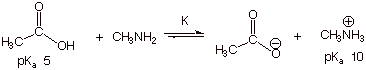(1). Qualitative Criterion: [1 pt]

The stronger acid is converted to the weaker acid. The lower pK­a corresponds to the stronger acid, so acetic acid is converted to the methylammonium ion. That is, the equilibrium lies to the right.

(2). Quantitative Criterion [3 pts]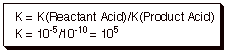B. Relative Acidities

1.    [4 pts] The equation for the acid dissociation of a general Bronsted acid, HA, in dilute aqueous solution is given below. Cite two general effects which are considered to influence the order of relative acidities of a series of Bronsted acids in which only the nature of A is varied. One of these effects should apply to a chemical species on the right hand side of the equation, and one to a species on the left hand side of the equation. Indicate in what sense (decrease or increase) each of these affects acidity and briefly explain why in each case.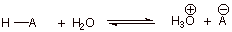a.     Anion Stability—The greater the stability (the lower the energy)of the anion, the more acidic is the Bronsted acid. That is because the anion is on the side of the equilibrium (the right side) which generates the hydronium ion.

b.    Bond Dissociation Energy (D) of HA—the lower the value of D (i.e., the weaker the bond), the higher is the acidity of HA. This is because the formation of hydronium ion requires the dissociation of HA (or because the less the stability of HA, the less favored is the left hand side  of the equilibrium).

2.    [1 pt] Which of these two general effects is normally dominant?

q      Anion Stability.

3.    [1 pts] In what specific type of case is the alternate general effect dominant?

q      When A is varied down a column or Group of the periodic table.

4.    [6 pts; 2 pts for each part] Stabilization of the relevant species on the right hand side of the above equation can be potentially achieved by means of at least four different specific effects. Name three of these effects and provide examples (one for each specific effect) in which each of these effects results in an important change in acidity.

a.     Name of specific effect #1 and example of the operation of this effect: [In any order]

Electronegativity: The order of acidities is H-Cl>H­2O>NH3>CH4 because the relative electronegativity order is Cl>O>N>C. Thus the chloride ion is more stable than hydroxide ion than the amide ion than the methyl carbanion.

b.    Name of specific effect #2 and example:

Hybridization: The order of acidities is ethyne>ethene>ethane, because the conjugate base of ethyne has its unshared pair in an sp orbital which, being 50% s, is much lower in energy, and more stable than the conjugate base of ethene, which has the unshared pair in an sp2 AO (only 33% s), and the conjugate base of ethane, which has the unshared pair in an sp3 AO (only 25% s).

c.     Name of specific effect #3 and example:

Resonance: The order of acidities is acetic acid>ethanol, because, although the negative charge is on oxygen in the conjugate base of both acids, the acetate ion is strongly resonance stabilized, because the negative charge is delocalized over two oxygens.

Inductive Effects: Only three effects are required in this question, but a fourth possibility is the inductive effect. For example, chloroacetic acid is a stronger acid than acetic acid, because the C-Cl dipole in the conjugate acid of chloroacetic acid places a partial positive charge on the carbon, which is closer to the sites of the negative charge than is the partial negative charge on the chlorine. This results in an electrostatic attraction between the negative charge of the anion and the partial positive charge on carbon, which results in stabilization of the chloroacetate anion.

### II. Stereochemistry

#### A. Number of Isomers

1.    [2 pt each = 4 pts] Indicate with an asterisk any stereogenic centers which are present in the compounds below. Then indicate how many stereoisomers there are in each case. Provide a specific rule for deriving the number of stereoisomers there are, and explain the application of the rule to each case.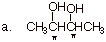q      There are three stereoisomers. There are two stereocenters (the two front carbons), but they are equivalent (have all of the same substituents

q      The n+1 rule, where n is the number of equivalent stereocenters. Since n = 2, here, there are three stereoisomers.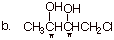q      There are two stereocenters, and they are non-equivalent

q      The 2n Rule applies, where n is the number of non-equivalent stereocenters. Since n = 2 here, there are four stereoisomers.

2.    [4 pts] Provide Newman projection structures for each stereoisomer of the following molecule (2,3-dibromobutane), and indicate the relationships between these isomers (what type of isomerism?). Assuming that the structure drawn below is the (S,S) isomer, designate the R,S names of each stereoisomer.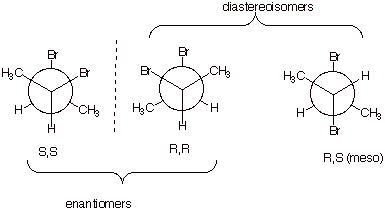3.    [3 pts] Specify the number of stereoisomers of the following Fischer structure, and explain your reasoning. Draw the Fischer structure of the enantiomer of this structure in the space provided to the right of it.

There are four non-equivalent stereocenters, so there are 24 or 16 stereoisomers.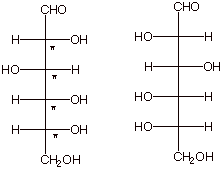### III. Alkenes: Nomenclature and Stability

A.  IUPAC Nomenclature

1. [3 pts] Provide the correct and complete IUPAC name for the following alkene, including any appropriate E/Z designation: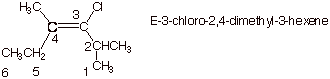2. [2 pts] Indicate the degree of substitution of this alkene (mono-, di-, tri-, etc), and explain how you derived your answer.

q      Trisubstituted alkene. Alkene  carbon 4 has methyl and ethyl substituents and alkene carbon 3 has just one substituent.

3. [3 pts] Provide the correct structure of the alkene which has the IUPAC name cis-1-ethyl-3-(1,1-dimethylpropyl)cyclohexane.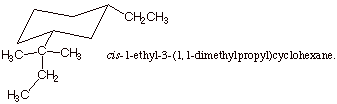B. Alkene Stability.

1.    [4 pts] The hydrogenation of both 1-butene and trans-2-butene gives the same product, butane. Illustrate, via an energy level diagram, the relative energies of these two alkenes, their energy difference in kcal/mol, and explain, using your diagram, how heats of hydrogenation reveal the exact magnitude of the difference in energies of these two alkenes.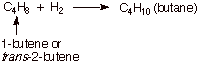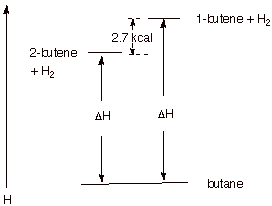Since both alkenes hydrogenate to the same product, any difference in their heats of hydrogenation must reflect energy differences in the alkenes (hydrogen is a common reactant, also). Since 1-butene has the higher heat of hydrogenation, it is the less stable (higher energy) alkene. The difference is ca. 2.7 kcal/mol (plus or minus 0.2 kcal acceptable).

2.    [3 pts] Provide the same kind of energy level diagram comparison for the hydrogenations of cis-2-butene and trans-2-butene. Which alkene is more stable and by how much? What is the basis for this difference in stability?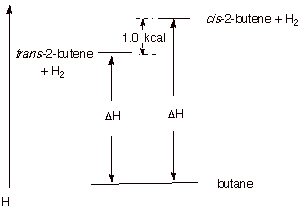q      Trans-2-butene is more stable by 1.0 kcal/mol.

q      This is because the cis isomer is destabilized a by steric interaction between the proximate methyl groups.

C. Pi Bonding In Alkenes.

1. [3 pts] Illustrate by means of Newman projection structures, and also showing the relevant overlap of 2p orbitals in pi bonding, what happens when rotation around the C-C bond of cis-2-butene occurs to ultimately give trans-2-butene. Is this easy or difficult? How much of the pi bond remains at the 90 degree angle of rotation? Explain, using an illustration.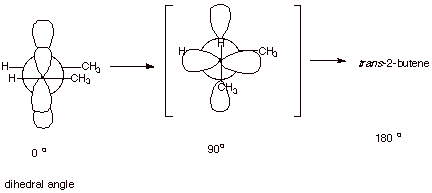q      This is very difficult because the overlap between the 2p orbitals rapidly decreases as the angle departs from zero degrees, thus sharply diminishing pi bonding.

q      At the 90 degree angle, the overlap is exactly zero, i.e., the pi bond is entirely broken. This is because the positive overlap just exactly balances the negative overlap.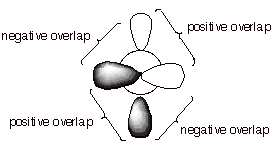IV. Reaction Mechanisms: General; Carbocation Intermediates

A.  Reaction Path Diagrams

1. Consider the following generalized two step reaction: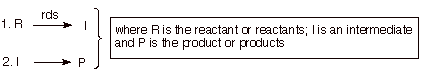a.     [5 pts] Sketch a reaction path diagram which realistically could represent such a reaction, indicating the position on the reaction coordinate of R, I, and P and any transition states (TS’s). Designate the TS’s as TS1 and TS2.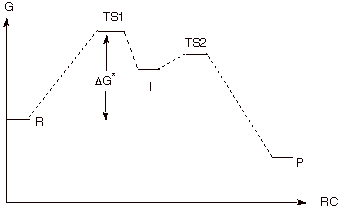b.    [1 pts] Indicate on the same diagram the energy quantity which controls the rate of the reaction.

c.     See above diagram.

d.    [4 pt] Define the term “rate-determining step”. Indicate two specific conditions which must be fulfilled for a step to be rigorously rate-determining. For each condition, point out on your reaction diagram how this condition is satisfied.

q      Rds is a step of a reaction, the rate of which is equal to the rate of the overall reaction (product formation).

q      Necessary conditions: (1) The intermediate must never go back to reactants but always on to products (2) The intermediate must not build up in concentration, but go rapidly to products. Condition 1 is met on the diagram by the illustration that the barrier preventing I from going back to reactants is larger than the barrier separating I from the product. Ccondition 2 is met by the circumstance that the activation energy for going on to products is very small.

e.     [2 pts] Explain why a step which is reversible (even partially), no matter how slow it may be, cannot be rate-determining.

q      If the step is reversible, some I is returning to reactants. Therefore the rate of product formation is less than the rate of formation of I in the first step.

C. Carbocation Intermediates

1.    [4 pts] Starting with the ground state of positively charged carbon atoms (the atomic number of carbon is, of course, 6), and using the linear format for representing ground states, show in a stepwise fashion how the hybridization state of carbon which is present in a carbocation center is derived. What is the name (designation) of this hybridization state?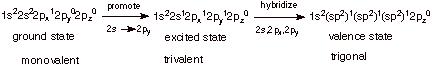2.    [2 pts] Which of the carbocations depicted below, the methyl carbocation or the ethyl carbocation is more thermodynamically stable and by how much (approximately, in solution)?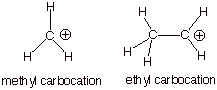The ethyl carbocation is the more stable cation by ca. 15 kcal/mol in solution [much more than this in the gas phase].

3.    [3 pts] Provide a resonance theoretical description of the resonance stabilization which exists in one of these carbocations. What specific sub-type of resonance effect is this?

q      This is called “hyperconjugation”.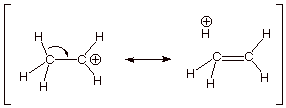4.    [2 pts] Using an orbital picture to illustrate the appropriate overlap of atomic orbitals, illustrate the overlap in the ethyl carbocation which gives rise to the delocalization and stabilization of the carbocation.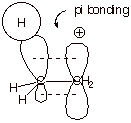3.    [2 pts] Provide the appropriate name for and a depiction of the other type of effect by which methyl or other alkyl groups stabilize a carbocation center.

q      The inductive effect.

q      The positive charge on carbon makes it electronegative, and it creates (induces) a dipole in the C-C bond, which stabilizes the positive charge.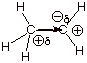4.    [3 pts] Indicate which of the carbocations illustrated below is the more stable one and explain why.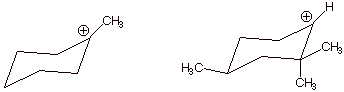q      The one on the left is more stable, because it is tertiary, while the one on the right is secondary. Each successive alkyl group substitution on the carbocation center increases stability.

V. Mechanism of H-Cl Additions to Alkenes

A. The Reaction Mechanism.

1. [4 pts] Write the detailed reaction mechanism for the addition of H-Cl to propene, employing all of the conventions required in  this course. Be certain to write each step of the mechanism on a separate, numbered line, to use electron flow arrows, and to designate any rate-determining steps or equilibrium steps.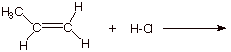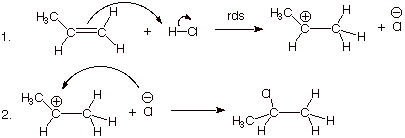2. [2 pts] If one of the steps of this mechanism is rate-determining, explain in energetic terms why it is this step in particular which is rate-determining and not the other step.

q      The first step is rate-determining because two bonds are broken in this step and only one formed, while in the seoncd step no bonds are broken and a bond is formed. The first step requires extensive energy input.

3. [4 pts] Draw a reaction path diagram for this reaction, specifying on your diagram the structures of I and P.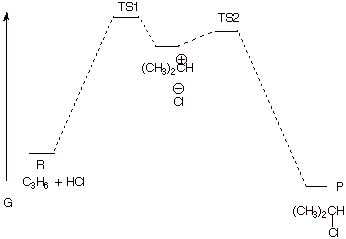B. Transition State Models.

1. [6 pts] Provide a resonance theoretical treatment of the transition state for the rds of this reaction, summarize this as a DL/PC (dotted line/partial charge) structure, and characterize the TS as specifically as you can (without yet applying the Hammond Principle). Do not merely write down the DL/PC model!! You must derive it using resonance theory!!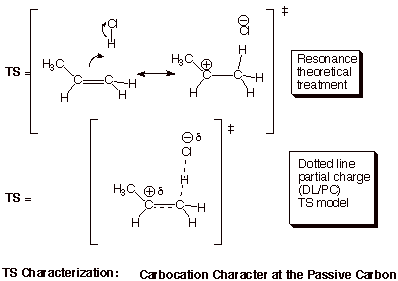2. [2 pts] State the Hammond Principle in its most concise form, and use it to further refine your TS model, explaining the steps in your logic.

q      The TS of a reaction step more closely resembles the higher energy partner (R or P) or that step.

q      Since, as stated earlier, the first step is highly endergonic (endothermic as well), the higher energy partner is the product of that step, which is the carbocation.

q      Therefore, the TS of this rds closely resembles the carbocation. We can say that the TS has “extensive (or highly developed) carbocation character at the passive carbon”.

C. Selectivity.

Consider, now, the hypothetical addition of H-Cl to propene in the opposite orientational sense, i.e., in which the product is 1-chloropropane.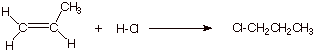1.    [4 pts] Modify the TS model you previously derived for the addition of HCl to propene to accommodate the different orientational sense. Compare the two models, side by side (Method of Competing TS’s), and provide a refined characterization of each TS model. Use this characterization to explain why the addition of HCl does not give 1-chloropropane.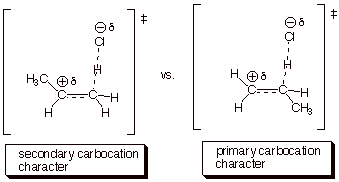a.     The second TS is less favorable than the first, because primary carbocation character is less favorable than secondary carbocation character. The second TS would lead to 1-chloropropane.

2.    [2 pts] Explain how the Hammond Principle and its application to the rds of this reaction allow us to rationalize the degree of selectivity of the HCl addition.

a.     The Hammond Principle application indicated that there was extensive carbocation character. The greater the extent of carbocation character, the greater the sensitivity to the difference in stability of a secondary vs a primary carbocation.

b.    The Hammond Principle therefore predicts a high degree of selectivity. (If there was little carbocation character, there would be relatively little selectivity).

3.    [1 pt] What type of selectivity is this (a formal name for the selectivity type)?

q      Regioselectivity (or regiospecificity)

5. [1 pt] What is the symbolic mechanistic designation of this reaction?